# 计算数学表达式（第二部分）。 普拉特和分流场解析器

27 十月 2020, 14:27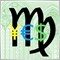0
1 040

### 解析器采用运算符优先级

```  class ExpressionPrecedence: public AbstractExpressionProcessor<Promise *>
{
protected:
static uchar prefixes;
static uchar infixes;

static ExpressionPrecedence epinit;

static void initPrecedence()
{
// 分组
prefixes['('] = 9;

// 一元运算符
prefixes['+'] = 9;
prefixes['-'] = 9;
prefixes['!'] = 9;

// 标识符
prefixes['_'] = 9;
for(uchar c = 'a'; c <= 'z'; c++)
{
prefixes[c] = 9;
}

// 数字
prefixes['.'] = 9;
for(uchar c = '0'; c <= '9'; c++)
{
prefixes[c] = 9;
}

// 运算符
// infixes['('] = 9; // 此处不将括号用作“函数调用”运算符
infixes['*'] = 8;
infixes['/'] = 8;
infixes['%'] = 8;
infixes['+'] = 7;
infixes['-'] = 7;
infixes['>'] = 6;
infixes['<'] = 6;
infixes['='] = 5;
infixes['!'] = 5;
infixes['&'] = 4;
infixes['|'] = 4;
infixes['?'] = 3;
infixes[':'] = 2;
infixes[','] = 1; // 参数列表定界符
}

ExpressionPrecedence(const bool init)
{
initPrecedence();
}

public:
ExpressionPrecedence(const string vars = NULL): AbstractExpressionProcessor(vars) {}
ExpressionPrecedence(VariableTable &vt): AbstractExpressionProcessor(vt) {}
};

static uchar ExpressionPrecedence::prefixes = {0};
static uchar ExpressionPrecedence::infixes = {0};
static ExpressionPrecedence ExpressionPrecedence::epinit(true);

```

```  class ExpressionPrecedence: public AbstractExpressionProcessor<Promise *>
{
protected:
...
{
int i = 1;
while(_index + i < _length && isspace(_expression[_index + i])) i++;
if(_index + i < _length)
{
return _expression[_index + i];
}
return 0;
}

void _matchNext(ushort c, string message, string context = NULL)
{
{
_nextToken();
}
else if(!_failed) // prevent chained errors
{
error(message, context);
}
}
...
};

```

### 普拉特解析器 (ExpressionPratt)

```  class ExpressionPratt: public ExpressionPrecedence
{
public:
ExpressionPratt(const string vars = NULL): ExpressionPrecedence(vars) { helper = new ExpressionHelperPromise(&this); }
ExpressionPratt(VariableTable &vt): ExpressionPrecedence(vt) { helper = new ExpressionHelperPromise(&this); }

virtual Promise *evaluate(const string expression) override
{
Promise::environment(&this);
AbstractExpressionProcessor<Promise *>::evaluate(expression);
if(_length > 0)
{
return parseExpression();
}
return NULL;
}

```

```      virtual Promise *parseExpression(const int precedence = 0)
{
if(_failed) return NULL; // 出错时截断子表达式

_nextToken();
if(prefixes[(uchar)_token] == 0)
{
this.error("Can't parse " + ShortToString(_token), __FUNCTION__);
return NULL;
}

Promise *left = _parsePrefix();

while((precedence < infixes[_token]) && !_failed)
{
left = _parseInfix(left, infixes[(uchar)_token]);
}

return left;
}

```

_parseInfix 方法的特定功能是，当前的 Promise (left) 对象会在第一个参数中被传递到内部，成为子表达式的一部分，而在该方法的第二个参数里设置允许的最小操作优先级，作为当前中缀令牌的优先级。 该方法返回依据整个子表达式生成的新 Promise 对象。 该对象保存在相同的变量中（之前的 Promise 引用，则会通过新对象的引用字段以某种方式提供）。

_parsePrefix 方法期待来自许可前缀的当前令牌，并利用 “switch” 进行处理。 对于开头的括号 '('，调用已熟知的方法 parseExpression 来计算嵌套表达式。 优先级参数则被省略，这意味着从最低的零优先级进行解析（因为它就像方括号中的单独表达式一样）。 一个 “helper” 对象用于 “!”，以便接收逻辑非得下一个片段。 它由 parseExpression 方法读取，但这次会将当前令牌的优先级传递给它。 这意味着取非的片段将在第一个符号之前以低于 “!” 的优先级结束。 例如，如果表达式为 “!a*b”，则 parseExpression 将在读取 “a” 变量后停止，因为乘法 “*” 的优先级低于取非 “!” 的优先级。 一元 '+' 和 '-' 以类似的方式处理，但在这种情况下不使用 “helper”。 对于 “+”，我们只需要让 parseExpression 读取子表达式。 对于 '-'，调用覆盖方法 “minus” 接收结果（您应当记得，结果是 Promise 对象）。

_parsePrefix 方法根据它们是否属于 “isalpha” 类别为所有其他符号排序。 假定字母是标识符的开头，数字或句点是数字的开头。 在所有其他情况下，该方法将返回 NULL。

```      Promise *_parsePrefix()
{
Promise *result = NULL;
switch(_token)
{
case '(':
result = parseExpression();
_match(')', ") expected!", __FUNCTION__);
break;
case '!':
result = helper._negate(parseExpression(prefixes[_token]));
break;
case '+':
result = parseExpression(prefixes[_token]);
break;
case '-':
result = -parseExpression(prefixes[_token]);
break;
default:
if(isalpha(_token))
{
string variable;

while(isalnum(_token))
{
variable += ShortToString(_token);
_nextToken();
}

if(_token == '(')
{
const string name = variable;
const int index = _functionTable.index(name);
if(index == -1)
{
error("Function undefined: " + name, __FUNCTION__);
return NULL;
}

const int arity = _functionTable[index].arity();
if(arity > 0 && _lookAhead() == ')')
{
error("Missing arguments for " + name + ", " + (string)arity + " required!", __FUNCTION__);
return NULL;
}

Promise *params[];
ArrayResize(params, arity);
for(int i = 0; i < arity; i++)
{
params[i] = parseExpression(infixes[',']);
if(i < arity - 1)
{
if(_token != ',')
{
_match(',', ", expected (param-list)!", __FUNCTION__);
break;
}
}
}

_match(')', ") expected after " + (string)arity + " arguments!", __FUNCTION__);

result = helper._call(index, params);
}
else
{
return helper._variable(variable); // 获取索引（如果找不到）- 选择使用 nan 保留名称
}
}
else // 暗示数字，必须为数字
{
string number;
{
return helper._literal(number);
}
}
}
return result;
}

```

_parseInfix 方法期望当前令牌是允许的中缀之一。 甚至，在第一个参数中，它接收已被读入 Promise *left 对象的左侧操作数。 第二个参数指定所要解析令牌的最小优先级。 一旦遇到优先级较低的内容，该子表达式即被视为结束。 _parseInfix 的目的是采用 'precedence' 调用 parseExpression，以便读取正确的操作数，然后，我们可以为二元操作相应的中缀创建 Promise 对象。

```      Promise *_parseInfix(Promise *left, const int precedence = 0)
{
Promise *result = NULL;
const ushort _previous = _token;
switch(_previous)
{
case '*':
case '/':
case '%':
case '+':
case '-':
result = new Promise((uchar)_previous, left, parseExpression(precedence));
break;
case '>':
case '<':
{
_nextToken();
result = new Promise((uchar)(_previous == '<' ? '{' : '}'), left, parseExpression(precedence));
}
else
{
result = new Promise((uchar)_previous, left, parseExpression(precedence));
}
break;
case '=':
case '!':
_matchNext('=', "= expected after " + ShortToString(_previous), __FUNCTION__);
result = helper._isEqual(left, parseExpression(precedence), _previous == '=');
break;
case '&':
case '|':
_matchNext(_previous, ShortToString(_previous) + " expected after " + ShortToString(_previous), __FUNCTION__);
result = new Promise((uchar)_previous, left, parseExpression(precedence));
break;
case '?':
{
Promise *truly = parseExpression(infixes[':']);
if(_token != ':')
{
_match(':', ": expected", __FUNCTION__);
}
else
{
Promise *falsy = parseExpression(infixes[':']);
if(truly != NULL && falsy != NULL)
{
result = helper._ternary(left, truly, falsy);
}
}
}
case ':':
case ',': // 跳过
break;
default:
error("Can't process infix token " + ShortToString(_previous));

}
return result;
}

```

### 字节码生成

```  struct ByteCode
{
uchar code;
double value;
int index;

ByteCode(): code(0), value(0.0), index(-1) {}
ByteCode(const uchar c): code(c), value(0.0), index(-1) {}
ByteCode(const double d): code('n'), value(d), index(-1) {}
ByteCode(const uchar c, const int i): code(c), value(0.0), index(i) {}

string toString() const
{
return StringFormat("%s %f %d", CharToString(code), value, index);
}
};

```

“code” 字段包含命令的本质（该值对应于 Promise 代码），“value” 字段包含一个数字（常量），而 “index” 字段包含在变量/函数表中的索引。

RPN 非常适合字节码，因为它能用堆栈轻松实现计算。 当程序在输入流中“看到”数字或变量引用时，它会把该值压入堆栈。 如果在输入流中遇到运算符或函数，则程序会从堆栈中弹出所需数量的值，用这些值执行指定的操作，然后将结果压回堆栈之中。 在该过程结束时，表达式评估结果会是堆栈上保留得唯一数字。

```  class Promise
{
...
public:
void exportToByteCode(ByteCode &codes[])
{
if(left) left.exportToByteCode(codes);
const int truly = ArraySize(codes);

if(code == '?')
{
ArrayResize(codes, truly + 1);
codes[truly].code = code;
}

if(right) right.exportToByteCode(codes);
const int falsy = ArraySize(codes);
if(last) last.exportToByteCode(codes);
const int n = ArraySize(codes);

if(code != '?')
{
ArrayResize(codes, n + 1);
codes[n].code = code;
codes[n].value = value;
codes[n].index = index;
}
else // (code == '?')
{
codes[truly].index = falsy; // 跳过 true 分支
codes[truly].value = n;     // 跳过两个分支
}
}
...
};

```

```    ExpressionPratt e(vars);
Promise *p = e.evaluate(expr);

ByteCode codes[];
p.exportToByteCode(codes);

for(int i = 0; i < ArraySize(codes); i++)
{
Print(i, "] ", codes[i].toString());
}

```

```  #define STACK_SIZE 100

// 堆栈模仿
#define push(S,V,N) S[N++] = V
#define pop(S,N) S[--N]
#define top(S,N) S[N-1]

class Promise
{
...
public:
static double execute(const ByteCode &codes[], VariableTable *vt = NULL, FunctionTable *ft = NULL)
{
if(vt) variableTable = vt;
if(ft) functionTable = ft;

double stack[]; int ssize = 0; ArrayResize(stack, STACK_SIZE);
int jumps[]; int jsize = 0; ArrayResize(jumps, STACK_SIZE / 2);
const int n = ArraySize(codes);
for(int i = 0; i < n; i++)
{
if(jsize && top(jumps, jsize) == i)
{
--jsize; // 快速 "弹 & 压 （pop & drop）"
i = pop(jumps, jsize);
continue;

}
switch(codes[i].code)
{
case 'n': push(stack, codes[i].value, ssize); break;
case 'v': push(stack, variableTable[codes[i].index], ssize); break;
case 'f':
{
IFunctor *ptr = functionTable[codes[i].index];
double params[]; ArrayResize(params, ptr.arity()); int psize = 0;
for(int j = 0; j < ptr.arity(); j++)
{
push(params, pop(stack, ssize), psize);
}
ArrayReverse(params);
push(stack, ptr.execute(params), ssize);
}
break;
case '+': push(stack, pop(stack, ssize) + pop(stack, ssize), ssize); break;
case '-': push(stack, -pop(stack, ssize) + pop(stack, ssize), ssize); break;
case '*': push(stack, pop(stack, ssize) * pop(stack, ssize), ssize); break;
case '/': push(stack, Promise::safeDivide(1, pop(stack, ssize)) * pop(stack, ssize), ssize); break;
case '%':
{
const double second = pop(stack, ssize);
const double first = pop(stack, ssize);
push(stack, fmod(first, second), ssize);
}
break;
case '!': push(stack, (double)(!pop(stack, ssize)), ssize); break;
case '~': push(stack, (double)(-pop(stack, ssize)), ssize); break;
case '<':
{
const double second = pop(stack, ssize);
const double first = pop(stack, ssize);
push(stack, (double)(first < second), ssize);
}
break;
case '>':
{
const double second = pop(stack, ssize);
const double first = pop(stack, ssize);
push(stack, (double)(first > second), ssize);
}
break;
case '{':
{
const double second = pop(stack, ssize);
const double first = pop(stack, ssize);
push(stack, (double)(first <= second), ssize);
}
break;
case '}':
{
const double second = pop(stack, ssize);
const double first = pop(stack, ssize);
push(stack, (double)(first >= second), ssize);
}
break;
case '&': push(stack, (double)(pop(stack, ssize) && pop(stack, ssize)), ssize); break;
case '|':
{
const double second = pop(stack, ssize);
const double first = pop(stack, ssize);
push(stack, (double)(first || second), ssize); // 顺序很重要
}
break;
case '`': push(stack, _precision < fabs(pop(stack, ssize) - pop(stack, ssize)), ssize); break;
case '=': push(stack, _precision > fabs(pop(stack, ssize) - pop(stack, ssize)), ssize); break;
case '?':
{
const double first = pop(stack, ssize);
if(first) // true
{
push(jumps, (int)codes[i].value, jsize); // 直至结束所在
push(jumps, codes[i].index, jsize);      // 我们从真实的终点跳跃
}
else // false
{
i = codes[i].index - 1; // 由于即将出现 ++，因此需要 -1
}
}
break;
default:
Print("Unknown byte code ", CharToString(codes[i].code));
}
}
return pop(stack, ssize);
}
...
};

```

```    ExpressionPratt e(vars);
Promise *p = e.evaluate(expr);

ByteCode codes[];
p.exportToByteCode(codes);
double r = Promise::execute(codes);

```

### 分流场解析器 (ExpressionShuntingYard)

```  在循环中读取表达式的下一个标记（直到表达式结束）
如果令牌是一元操作，则将其保存在堆栈中
如果是一个数字，则将其写入字节码
如果是一个变量，则将其索引写入字节码
如果它是一个函数标识符，则将其索引保存在堆栈上
如果令牌是中缀运算符
只要 “(” 不在堆栈顶部，且堆栈顶部的运算符优先级 >= 当前运算符优先级，或函数位于顶部
将堆栈顶部推入输出字节码
将运算符保存到堆栈上
如果令牌是 “(”，则将其保存在堆栈中
如果令牌是 ')'
只要堆栈顶不是 '('
将堆栈顶部推入输出字节码
如果堆栈顶是 '('，删除并废弃
如果堆栈上还有遗留标记，则按顺序将它们移动到输出字节码中

```

```  class ExpressionShuntingYard: public ExpressionPrecedence
{
public:
ExpressionShuntingYard(const string vars = NULL): ExpressionPrecedence(vars) { }
ExpressionShuntingYard(VariableTable &vt): ExpressionPrecedence(vt) { }

bool convertToByteCode(const string expression, ByteCode &codes[])
{
Promise::environment(&this);
AbstractExpressionProcessor<Promise *>::evaluate(expression);
if(_length > 0)
{
exportToByteCode(codes);
}
return !_failed;
}

protected:
template<typename T>
static void _push(T &stack[], T &value)
{
const int n = ArraySize(stack);
ArrayResize(stack, n + 1, STACK_SIZE);
stack[n] = value;
}

void exportToByteCode(ByteCode &output[])
{
ByteCode stack[];
int ssize = 0;
string number;
uchar c;

ArrayResize(stack, STACK_SIZE);

const int previous = ArraySize(output);

while(_nextToken() && !_failed)
{
if(_token == '+' || _token == '-' || _token == '!')
{
if(_token == '-')
{
_push(output, ByteCode(-1.0));
push(stack, ByteCode('*'), ssize);
}
else if(_token == '!')
{
push(stack, ByteCode('!'), ssize);
}
continue;
}

number = "";
{
_push(output, ByteCode(StringToDouble(number)));
}

if(isalpha(_token))
{
string variable;
while(isalnum(_token))
{
variable += ShortToString(_token);
_nextToken();
}
if(_token == '(')
{
push(stack, ByteCode('f', _functionTable.index(variable)), ssize);
}
else // 变量名
{
int index = -1;
if(CheckPointer(_variableTable) != POINTER_INVALID)
{
index = _variableTable.index(variable);
if(index == -1)
{
{
_push(output, ByteCode('v', index));
error("Unknown variable is NaN: " + variable, __FUNCTION__, true);
}
else
{
error("Unknown variable : " + variable, __FUNCTION__);
}
}
else
{
_push(output, ByteCode('v', index));
}
}
}
}

if(infixes[_token] > 0) // 运算符，包括最低有效值 '?'
{
while(ssize > 0 && isTop2Pop(top(stack, ssize).code))
{
_push(output, pop(stack, ssize));
}

if(_token == '?' || _token == ':')
{
if(_token == '?')
{
const int start = ArraySize(output);
_push(output, ByteCode((uchar)_token));
exportToByteCode(output); // 子表达式为真，_token 已经改变了
if(_token != ':')
{
error("Colon expected, given: " + ShortToString(_token), __FUNCTION__);
break;
}
output[start].index = ArraySize(output);
exportToByteCode(output); // 子表达式为假，_token 已经改变了
output[start].value = ArraySize(output);
if(_token == ':')
{
break;
}
}
else
{
break;
}
}
else
{
if(_token == '>' || _token == '<')
{
{
push(stack, ByteCode((uchar)(_token == '<' ? '{' : '}')), ssize);
_nextToken();
}
else
{
push(stack, ByteCode((uchar)_token), ssize);
}
}
else if(_token == '=' || _token == '!')
{
{
push(stack, ByteCode((uchar)(_token == '!' ? '`' : '=')), ssize);
_nextToken();
}
}
else if(_token == '&' || _token == '|')
{
_matchNext(_token, ShortToString(_token) + " expected after " + ShortToString(_token), __FUNCTION__);
push(stack, ByteCode((uchar)_token), ssize);
}
else if(_token != ',')
{
push(stack, ByteCode((uchar)_token), ssize);
}
}
}

if(_token == '(')
{
push(stack, ByteCode('('), ssize);
}
else if(_token == ')')
{
while(ssize > 0 && (c = top(stack, ssize).code) != '(')
{
_push(output, pop(stack, ssize));
}
if(c == '(') // 除非是子表达式，否则必须为 true（那么 “c” 可以为 0）
{
ByteCode disable_warning = pop(stack, ssize);
}
else
{
if(previous == 0)
{
error("Closing parenthesis is missing", __FUNCTION__);
}
return;
}
}
}

while(ssize > 0)
{
_push(output, pop(stack, ssize));
}
}

bool isTop2Pop(const uchar c)
{
return (c == 'f' || infixes[c] >= infixes[_token]) && c != '(' && c != ':';
}
};

```

```  ExpressionShuntingYard sh;

ByteCode codes[];
bool success = sh.convertToByteCode("x + y", codes);
if(success)
{
sh.variableTable().assign("x=10;y=20");
double r = Promise::execute(codes);
}

```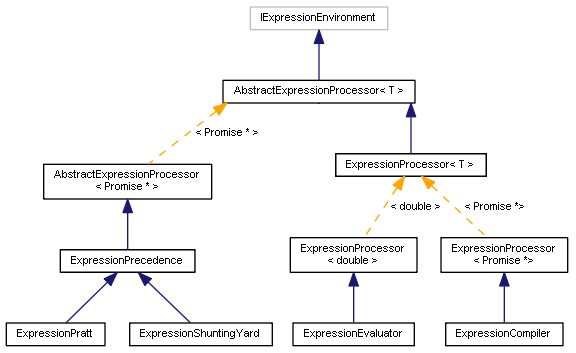### 在表达式中嵌入指标作为函数

```  class IndicatorFunc: public AbstractFunc
{
public:
IndicatorFunc(const string n, const int a = 1): AbstractFunc(n, a)
{
// 单参数是柱线数量，
// 两个参数是柱线数量和缓冲区索引
}
static IndicatorFunc *create(const string name);
};

```

Metatrader 5 中指标的具体特点是，它们还需要两个应用阶段：首先我们需要创建指标（为了获取其描述），然后从中请求数据。 在表达式处理的上下文中，第一步应该在解析期间执行，第二步应该在求值期间执行。 由于创建指标时需要指定所有参数，因此它们必须以名称实现，取代通过函数参数中传递。 例如，如果我们采用参数（period，method，price_type）创建 “iMA” 函数，那么在解析阶段我们只会收到它的名称，而参数的定义会推迟到执行阶段，这时创建指标已经太晚了（因为我们在这个阶段应该从指标中读取数据）。

MAIndicatorFunc 类依据参数所需的相应名称创建指标实例。

```  class MAIndicatorFunc: public IndicatorFunc
{
protected:
const int handle;

public:
MAIndicatorFunc(const string n, const int h): IndicatorFunc(n), handle(h) {}

~MAIndicatorFunc()
{
IndicatorRelease(handle);
}

static MAIndicatorFunc *create(const string name) // SMA_OPEN_10(0)
{
string parts[];
if(StringSplit(name, '_', parts) != 3) return NULL;

ENUM_MA_METHOD m = -1;
ENUM_APPLIED_PRICE t = -1;

static string methods[] = {"SMA", "EMA", "SMMA", "LWMA"};
for(int i = 0; i < ArraySize(methods); i++)
{
if(parts == methods[i])
{
m = (ENUM_MA_METHOD)i;
break;
}
}

static string types[] = {"NULL", "CLOSE", "OPEN", "HIGH", "LOW", "MEDIAN", "TYPICAL", "WEIGHTED"};
for(int i = 1; i < ArraySize(types); i++)
{
if(parts == types[i])
{
t = (ENUM_APPLIED_PRICE)i;
break;
}
}

if(m == -1 || t == -1) return NULL;

int h = iMA(_Symbol, _Period, (int)StringToInteger(parts), 0, m, t);
if(h == INVALID_HANDLE) return NULL;

return new MAIndicatorFunc(name, h);
}

double execute(const double &params[]) override
{
const int bar = (int)params;
double result = {0};
if(CopyBuffer(handle, 0, bar, 1, result) != 1)
{
Print("CopyBuffer error: ", GetLastError());
}
return result;
}
};

```

“create” 工厂方法解析传递给它的名称，从名称中提取参数，并使用 “handle” 创建一个指标。 指标值是通过标准函子方法获得 — execute。

```  static IndicatorFunc *IndicatorFunc::create(const string name)
{
// TODO:支持更多指标类型，根据名称调度调用
return MAIndicatorFunc::create(name);
}

```

```  class FunctionTable: public Table<IFunctor *>
{
public:
...
#ifdef INDICATOR_FUNCTORS
virtual int index(const string name) override
{
int i = _table.getIndex(name);
if(i == -1)
{
i = _table.getSize();
IFunctor *f = IndicatorFunc::create(name);
if(f)
{
return i;
}
return -1;
}
return i;
}
#endif
};

```

### 测试脚本（ExpressParser）

```  Running 19 tests on ExpressionPratt* …
1 passed, ok: a > b ? b > c ? 1 : 2 : 3 = 3.0; expected = 3.0
2 passed, ok: 2 > 3 ? 2 : 3 > 4 ? 3 : 4 = 4.0; expected = 4.0
3 passed, ok: 4 > 3 ? 2 > 4 ? 2 : 4 : 3 = 4.0; expected = 4.0
4 passed, ok: (a + b) * sqrt(c) = 8.944271909999159; expected = 8.944271909999159
5 passed, ok: (b == c) > (a != 1.5) = 0.0; expected = 0.0
6 passed, ok: (b == c) >= (a != 1.5) = 1.0; expected = 1.0
7 passed, ok: (a > b) || sqrt(c) = 1.0; expected = 1.0
8 passed, ok: (!1 != !(b - c/2)) = 1.0; expected = 1.0
9 passed, ok: -1 * c == -sqrt(-c * -c) = 1.0; expected = 1.0
10 passed, ok: pow(2, 5) % 5 = 2.0; expected = 2.0
11 passed, ok: min(max(a,b),c) = 2.5; expected = 2.5
12 passed, ok: atan(sin(0.5)/cos(0.5)) = 0.5; expected = 0.5
13 passed, ok: .2 * .3 + .1 = 0.16; expected = 0.16
14 passed, ok: (a == b) + (b == c) = 0.0; expected = 0.0
15 passed, ok: -(a + b) * !!sqrt(c) = -4.0; expected = -4.0
16 passed, ok: sin ( max ( 2 * 1.5, 3 ) / 3 * 3.14159265359 ) = -2.068231111547469e-13; expected = 0.0
lookUpVariable error: Variable is undefined: _1c @ 7: 1 / _1c^
17 passed, er: 1 / _1c = nan; expected = nan
safeDivide error: Error : Division by 0! @ 15: 1 / (2 * b - c)^
18 passed, er: 1 / (2 * b - c) = inf; expected = inf
19 passed, ok: sqrt(b-c) = -nan(ind); expected = -nan(ind)
19 tests passed of 19
17 for correct expressions, 2 for invalid expressions

```

```  >>> Performance tests (timing per method)
Evaluation: 104572
Compilation: 25011
Pratt bytecode: 23738
Pratt: 24967
ShuntingYard: 23147

```

### 使用表达式来为智能交易系统设置信号（ExprBot）

```  #define INDICATOR_FUNCTORS
#include <ExpresSParserS/ExpressionCompiler.mqh>

input string SignalBuy = "EMA_OPEN_{Fast}(0)/EMA_OPEN_{Slow}(0) > 1 + Threshold";
input string SignalSell = "EMA_OPEN_{Fast}(0)/EMA_OPEN_{Slow}(0) < 1 - Threshold";
input string Variables = "Threshold=0.01";
input int Fast = 10;
input int Slow = 21;

```

```  ExpressionCompiler ecb(Variables), ecs(Variables);
Promise *p1, *p2;

```

```  int OnInit()
{
ecb.variableTable().set("Fast", Fast);
ecb.variableTable().set("Slow", Slow);
if(!ecb.success())
{
p1.print();
return INIT_FAILED;
}
ecs.variableTable().set("Fast", Fast);
ecs.variableTable().set("Slow", Slow);
p2 = ecs.evaluate(SignalSell, true);
if(!ecs.success())
{
Print("Syntax error in Sell signal:");
p2.print();
return INIT_FAILED;
}

return INIT_SUCCEEDED;
}

```

```  #define _Ask SymbolInfoDouble(_Symbol, SYMBOL_ASK)
#define _Bid SymbolInfoDouble(_Symbol, SYMBOL_BID)

void OnTick()
{
if(!isNewBar()) return;

bool sell = p2.resolve();

{
sell = false;
}

{
OrdersCloseAll(_Symbol, OP_SELL);
{
}
}
else if(sell)
{
if(OrdersTotalByType(_Symbol, OP_SELL) == 0)
{
OrderSend(_Symbol, OP_SELL, Lot, _Bid, 100, 0, 0);
}
}
else
{
OrdersCloseAll();
}
}

```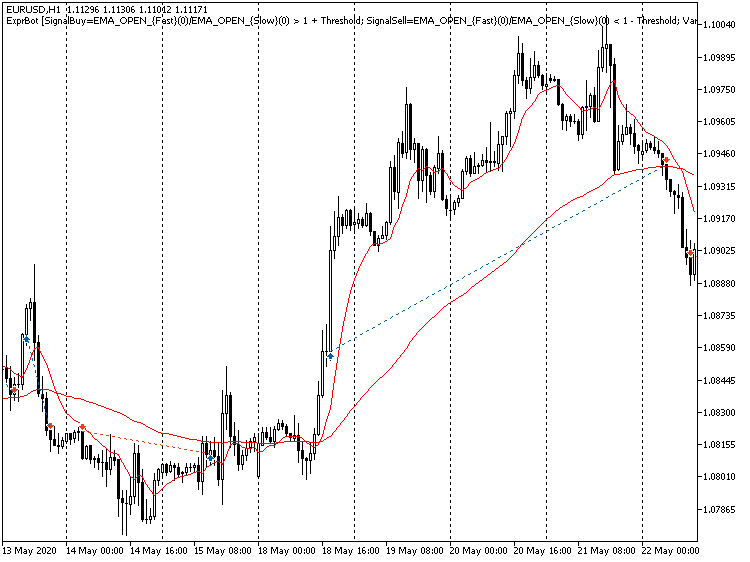### 结束语

parsers2.zip (40.45 KB)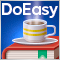DoEasy 函数库中的时间序列（第四十五部分）：多周期指标缓冲区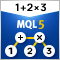计算数学表达式（第一部分）。 递归下降解析器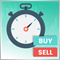快捷手动交易工具箱：持仓和挂单操控DoEasy 函数库中的时间序列（第四十六部分）：多周期、多品种指标缓冲区